# US20150207439A1 - Torque ripple reduction in switched reluctance motor drives - Google Patents

## Info

Publication number
US20150207439A1
US20150207439A1 US14/599,838 US201514599838A US2015207439A1 US 20150207439 A1 US20150207439 A1 US 20150207439A1 US 201514599838 A US201514599838 A US 201514599838A US 2015207439 A1 US2015207439 A1 US 2015207439A1
Authority
US
United States
Prior art keywords
torque
current
phase
tsf
ref
Prior art date
Legal status (The legal status is an assumption and is not a legal conclusion. Google has not performed a legal analysis and makes no representation as to the accuracy of the status listed.)
Granted
Application number
US14/599,838
Other versions
US9742320B2 (en
Inventor
Jin Ye
Current Assignee (The listed assignees may be inaccurate. Google has not performed a legal analysis and makes no representation or warranty as to the accuracy of the list.)
Enedym Inc
Original Assignee
McMaster University
Priority date (The priority date is an assumption and is not a legal conclusion. Google has not performed a legal analysis and makes no representation as to the accuracy of the date listed.)
Filing date
Publication date
Priority to US201461928693P priority Critical
Application filed by McMaster University filed Critical McMaster University
Priority to US14/599,838 priority patent/US9742320B2/en
Assigned to MCMASTER UNIVERSITY reassignment MCMASTER UNIVERSITY ASSIGNMENT OF ASSIGNORS INTEREST (SEE DOCUMENT FOR DETAILS). Assignors: YE, JIN
Assigned to MCMASTER UNIVERSITY reassignment MCMASTER UNIVERSITY CORRECTIVE ASSIGNMENT TO ADD SECOND INVENTOR NAME PREVIOUSLY RECORDED AT REEL: 035787 FRAME: 0609. ASSIGNOR(S) HEREBY CONFIRMS THE ASSIGNMENT. Assignors: EMADI, ALI, YE, JIN
Publication of US20150207439A1 publication Critical patent/US20150207439A1/en
Publication of US9742320B2 publication Critical patent/US9742320B2/en
Application granted granted Critical
Assigned to ENEDYM INC. reassignment ENEDYM INC. ASSIGNMENT OF ASSIGNORS INTEREST (SEE DOCUMENT FOR DETAILS). Assignors: MCMASTER UNIVERSITY
Active legal-status Critical Current

• 238000004088 simulation Methods 0.000 description 34
• 229910052802 copper Inorganic materials 0.000 description 30
• 239000010949 copper Substances 0.000 description 30
• 230000004907 flux Effects 0.000 description 28
• 230000001965 increased Effects 0.000 description 12
• 230000003247 decreasing Effects 0.000 description 8
• 230000001419 dependent Effects 0.000 description 7
• 238000004458 analytical method Methods 0.000 description 6
• 238000005457 optimization Methods 0.000 description 6
• 230000002829 reduced Effects 0.000 description 6
• 238000005070 sampling Methods 0.000 description 6
• 238000010586 diagram Methods 0.000 description 3
• 238000004519 manufacturing process Methods 0.000 description 3
• 230000000630 rising Effects 0.000 description 3
• 101700037397 MAX2 Proteins 0.000 description 2
• 241000156302 Porcine hemagglutinating encephalomyelitis virus Species 0.000 description 2
• 238000010276 construction Methods 0.000 description 2
• 238000009795 derivation Methods 0.000 description 2
• 230000004048 modification Effects 0.000 description 2
• 238000006011 modification reaction Methods 0.000 description 2
• 239000007787 solid Substances 0.000 description 2
• 238000004804 winding Methods 0.000 description 2
• 229910000831 Steel Inorganic materials 0.000 description 1
• 230000037098 T max Effects 0.000 description 1
• 230000035852 Tmax Effects 0.000 description 1
• 238000004364 calculation method Methods 0.000 description 1
• 238000006243 chemical reaction Methods 0.000 description 1
• 238000002485 combustion reaction Methods 0.000 description 1
• 230000000694 effects Effects 0.000 description 1
• 230000002708 enhancing Effects 0.000 description 1
• 238000011156 evaluation Methods 0.000 description 1
• 239000000446 fuel Substances 0.000 description 1
• 230000000670 limiting Effects 0.000 description 1
• 239000000696 magnetic material Substances 0.000 description 1
• 238000000034 method Methods 0.000 description 1
• 238000005065 mining Methods 0.000 description 1
• 239000000203 mixture Substances 0.000 description 1
• 230000036961 partial Effects 0.000 description 1
• 230000001681 protective Effects 0.000 description 1
• 239000010959 steel Substances 0.000 description 1

## Images

•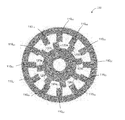•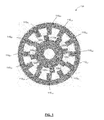•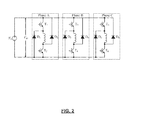•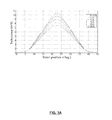•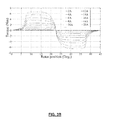•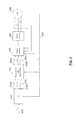•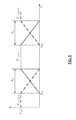•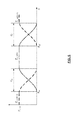••••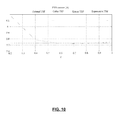••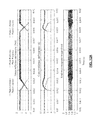•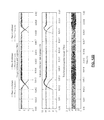•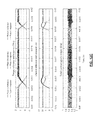•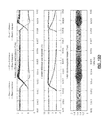•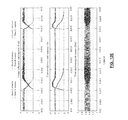•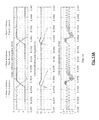•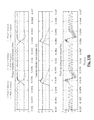•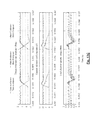•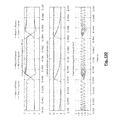•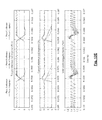•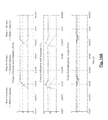•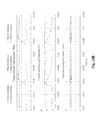•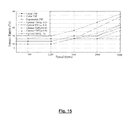•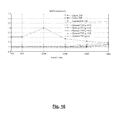•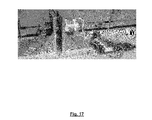••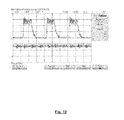••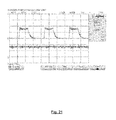## Classifications

• HELECTRICITY
• H02GENERATION; CONVERSION OR DISTRIBUTION OF ELECTRIC POWER
• H02PCONTROL OR REGULATION OF ELECTRIC MOTORS, ELECTRIC GENERATORS OR DYNAMO-ELECTRIC CONVERTERS; CONTROLLING TRANSFORMERS, REACTORS OR CHOKE COILS
• H02P6/00Arrangements for controlling synchronous motors or other dynamo-electric motors using electronic commutation dependent on the rotor position; Electronic commutators therefor
• H02P6/10Arrangements for controlling torque ripple, e.g. providing reduced torque ripple
• HELECTRICITY
• H02GENERATION; CONVERSION OR DISTRIBUTION OF ELECTRIC POWER
• H02PCONTROL OR REGULATION OF ELECTRIC MOTORS, ELECTRIC GENERATORS OR DYNAMO-ELECTRIC CONVERTERS; CONTROLLING TRANSFORMERS, REACTORS OR CHOKE COILS
• H02P25/00Arrangements or methods for the control of AC motors characterised by the kind of AC motor or by structural details
• H02P25/02Arrangements or methods for the control of AC motors characterised by the kind of AC motor or by structural details characterised by the kind of motor
• H02P25/08Reluctance motors
• H02P25/098Arrangements for reducing torque ripple
• H02P6/001
• H02P6/002
• BPERFORMING OPERATIONS; TRANSPORTING
• B62LAND VEHICLES FOR TRAVELLING OTHERWISE THAN ON RAILS
• B62DMOTOR VEHICLES; TRAILERS
• B62D5/00Power-assisted or power-driven steering
• B62D5/04Power-assisted or power-driven steering electrical, e.g. using an electric servo-motor connected to, or forming part of, the steering gear
• B62D5/0457Power-assisted or power-driven steering electrical, e.g. using an electric servo-motor connected to, or forming part of, the steering gear characterised by control features of the drive means as such
• B62D5/046Controlling the motor
• HELECTRICITY
• H02GENERATION; CONVERSION OR DISTRIBUTION OF ELECTRIC POWER
• H02PCONTROL OR REGULATION OF ELECTRIC MOTORS, ELECTRIC GENERATORS OR DYNAMO-ELECTRIC CONVERTERS; CONTROLLING TRANSFORMERS, REACTORS OR CHOKE COILS
• H02P6/00Arrangements for controlling synchronous motors or other dynamo-electric motors using electronic commutation dependent on the rotor position; Electronic commutators therefor
• H02P6/14Electronic commutators

## Abstract

A method for controlling a switched reluctance motor, the method comprising: receiving a reference torque Te ref; receiving an indication of a present rotor position θ for the switched reluctance motor; determining at least one of: a reference current ie ref(k−1) for a (k−1)th phase, a reference current ie ref(k) for a (k)th phase, and a reference current ie ref(k+1) for a (k+1)th phase; and outputting the determined at least one reference current to a current controller operatively coupled to the switched reluctance motor, wherein the determined at least one reference current is based on an objective function comprising the squares of phase current and derivatives of current reference.

## Description

FIELD
• Embodiments disclosed herein relate generally to torque sharing functions (TSF), and more particularly to TSFs for torque ripple reduction and efficiency improvement in switched reluctance motor (SRM) drives.
• BACKGROUND
• Hybrid vehicles (e.g. vehicles with more than one power source for supplying power to move the vehicle) may provide increased efficiency and/or increased fuel economy when compared to vehicles powered by a single internal combustion engine.
• Switched reluctance motor (SRM) drives are gaining interest in hybrid (HEV) and Plug-in Hybrid Electric Vehicle (PHEV) applications due to its simple and rigid structure, four-quadrant operation, and extended-speed constant-power range. However, SRM drives generally suffer from high commutation torque ripple, typically resulting from poor tracking precision of phase current, nonlinear inductance profiles, and nonlinear torque-current-rotor position characteristics.
• BRIEF DESCRIPTION OF THE DRAWINGS
• For a better understanding of the described embodiments and to show more clearly how they may be carried into effect, reference will now be made, by way of example, to the accompanying drawings in which:
• FIG. 1 is a schematic cross-section view of a switched reluctance motor;
• FIG. 2 is a circuit diagram for an asymmetric bridge converter in accordance with at least one example embodiment;
• FIG. 3A is an inductance profile for an example 12/8 SRM;
• FIG. 3B is a torque profile for an example 12/8 SRM;
• FIG. 4 is a schematic SRM torque control diagram in accordance with at least one example embodiment;
• FIG. 5 is a representative waveform for the linear TSF;
• FIG. 6 is a representative waveform for the cubic TSF;
• FIG. 7 shows calculated torque reference, current reference, flux linkage, and rate of change of flux linkage for linear, cubic, and exponential TSFs;
• FIG. 8 shows calculated torque reference, current reference, flux linkage, and rate of change of flux linkage for proposed TSFs in accordance with at least one example embodiment;
• FIG. 9 is a plot of maximum absolute rate of change of flux linkage for proposed TSFs in accordance with at least one example embodiment;
• FIG. 10 is a plot comparing RMS current values for linear, cubic, and exponential TSFs and proposed TSFs in accordance with at least one example embodiment;
• FIG. 11 is a plot comparing calculated and modeled torque profiles for proposed TSFs in accordance with at least one example embodiment;
• FIG. 12A shows simulation results for the linear TSF at 300 rpm (Tref=1.5 Nm);
• FIG. 12B shows simulation results for the cubic TSF at 300 rpm (Tref=1.5 Nm);
• FIG. 12C shows simulation results for the exponential TSF at 300 rpm (Tref=1.5 Nm);
• FIG. 12D shows simulation results for a proposed TSF (q=0.4) in accordance with at least one example embodiment at 300 rpm (Tref=1.5 Nm);
• FIG. 12E shows simulation results for a proposed TSF (q=1) in accordance with at least one example embodiment at 300 rpm (Tref=1.5 Nm);
• FIG. 13A shows simulation results for the linear TSF at 3,000 rpm (Tref=1.5 Nm);
• FIG. 13B shows simulation results for the cubic TSF at 3,000 rpm (Tref=1.5 Nm);
• FIG. 13C shows simulation results for the exponential TSF at 3,000 rpm (Tref=1.5 Nm);
• FIG. 13D shows simulation results for a proposed TSF (q=0.4) in accordance with at least one example embodiment at 3,000 rpm (Tref=1.5 Nm);
• FIG. 13E shows simulation results for a proposed TSF (q=1) in accordance with at least one example embodiment at 3,000 rpm (Tref=1.5 Nm);
• FIG. 14A shows simulation results for the linear TSF at 2,000 rpm (Tref=3 Nm);
• FIG. 14B shows simulation results for a proposed TSF (q=0.4) in accordance with at least one example embodiment at 2,000 rpm (Tref=3 Nm);
• FIG. 15 shows simulation results comparing torque ripple at different motor speeds for linear TSF, cubic TSF, exponential TSF, and proposed TSF (for q=0.2, q=0.4, q=0.6, q=0.8, and q=1) with Tref=1.5 Nm;
• FIG. 16 shows simulation results comparing RMS current at different motor speeds for linear TSF, cubic TSF, exponential TSF, and proposed TSF (for q=0.2, q=0.4, q=0.6, q=0.8, and q=1) with Tref=1.5 Nm;
• FIG. 17 shows experimental equipment used to test proposed TFSs;
• FIG. 18 shows experimental results for a proposed TSF (q=0.4) at 1,800 rpm (Udc=300 V, Tref=1.5 Nm);
• FIG. 19 shows experimental results for a proposed TSF (q=0.4) at 2,500 rpm (Udc=300 V, Tref=1.5 Nm);
• FIG. 20 shows experimental results for a proposed TSF (q=0.4) at 4,200 rpm (Udc=300 V, Tref=1.5 Nm); and
• FIG. 21 shows experimental results for a proposed TSF (q=0.4) at 2,300 rpm (Udc=300 V, Tref=3 Nm).
• Further aspects and features of the embodiments described herein will become apparent from the following detailed description taken together with the accompanying drawings. It should be understood, however, that the detailed description and the specific examples, while indicating preferred embodiments of the application, are given by way of illustration only, since various changes and modifications within the spirit and scope of the application will become apparent to those skilled in the art from this detailed description.
• DESCRIPTION OF EXAMPLE EMBODIMENTS
• Various systems or methods are described below to provide an example of an embodiment of each claimed invention. No embodiment described below limits any claimed invention and any claimed invention may cover systems and methods that differ from those described below. The claimed inventions are not limited to systems and methods having all of the features of any one system or method described below or to features common to multiple or all of the systems or methods described below. It is possible that a system or method described below is not an embodiment of any claimed invention. Any invention disclosed in a system or method described below that is not claimed in this document may be the subject matter of another protective instrument, for example, a continuing patent application, and the applicant(s), inventor(s) and/or owner(s) do not intend to abandon, disclaim, or dedicate to the public any such invention by its disclosure in this document.
• Switched reluctance motor (SRM) drives are gaining interest in hybrid (HEV) and Plug-in Hybrid Electric Vehicle (PHEV) applications due to its simple and rigid structure, four-quadrant operation, and extended-speed constant-power range. SRM drives have generally been considered to be reliable and cost effective in harsh environments due to, for example, the absence of windings and permanent magnet on the rotor. But SRM drives generally suffer from high commutation torque ripple, typically resulting from poor tracking precision of phase current, nonlinear inductance profiles, and nonlinear torque-current-rotor position characteristics.
• In typical TSFs, a torque reference is distributed among the motor phases, and the sum of the torque contributed by each phase is equal to the total reference torque. Then the reference phase current can be derived using the torque-current-rotor position characteristics of the particular SRM being driven.
• Conventional torque sharing functions (TSFs) for SRMs include linear, sinusoidal, quadratic, cubic, and exponential TSFs. The secondary objectives for the selection of a particular TSF include: i) minimizing the copper loss, and ii) enhancing the torque-speed capability.
• Selection of a torque sharing function will influence the phase current reference, and therefore the copper loss (which may also be referred to as the power loss) of the electric machine. Also, in order to track the torque reference, the effective rate of change of flux linkage should be minimized to extend the torque-speed range. Otherwise, with very limited DC-link voltage, phase current may be unable to track the reference perfectly during high speed, and therefore torque ripple increases with the rotating speed. However, the turn-off angle of conventional TSFs is typically defined only at the positive torque production area, which can lead to higher rate of change flux linkage with respect to rotor position. Thus, conventional TSFs generally have a relatively limited torque-ripple-free speed range.
• A TSF can be characterized as either offline or online. For example, some TSFs are tuned online by using estimated torque or speed feedback, and they typically require additional parameters.
• As disclosed herein, a new family of offline TSFs for torque ripple reduction and efficiency improvement of SRM drives over wide speed range has been developed. The objective function of the proposed TSFs is composed of two secondary objectives with a Tikhonov factor, in an effort to minimize the square of phase current (copper loss) and derivatives of current references (rate of change of flux linkage). The derivatives of current references are minimized to achieve better tracking precision of the torque reference during high speed, and therefore, to increase the torque-ripple-free speed range of the SRM.
• A family of proposed TSFs may be obtained with different Tikhonov factors by using the method of Lagrange multipliers. Performance of conventional TSFs and at least some of the family of proposed TSFs were compared in terms of efficiency and torque-speed performance over a wide speed range. Simulation and experimental results—using a 2.3 kW three-phase, 6000 rpm, 12/8 SRM—are provided herein to provide examples of the performance of the proposed family of TSFs. These results indicate that the proposed TSFs are capable of reducing both the torque ripple and copper loss of a SRM over a relatively wide speed range.
• In one broad aspect, there is provided a method for controlling a switched reluctance motor, the method comprising: receiving a reference torque Te ref; receiving an indication of a present rotor position θ for the switched reluctance motor; determining at least one of: a reference current ie ref(k−1) for a (k−1)th phase, a reference current ie ref(k) for a (k)th phase, and a reference current ie ref(k+1) for a (k+1)th phase; and outputting the determined at least one reference current to a current controller operatively coupled to the switched reluctance motor, wherein the determined at least one reference current is based on an objective function comprising the squares of phase current and derivatives of current reference.
• In some embodiments, the objective function comprises:
• $min   J = mi k - 1 2  ( θ ) + ni k 2  ( θ ) + s  [ i k - 1  ( θ ) - i k - 1  ( θ 0 ) Δθ ] 2 + t  [ i k  ( θ ) - i k  ( θ 0 ) Δθ ] 2$
• subject to:
• $1 2  ∂ L  ( θ , i k - 1 ) ∂ θ  i k - 1 2  ( θ ) + 1 2  ∂ L  ( θ , i k ) Δθ  i k 2  ( θ ) = T e —  ref ,  i k - 1 ≤ l max , and$ $i k ≤ l max ;$
• wherein:
• θ0 is an indication of a previous rotor position for the switched reluctance motor,
• ik−10) is a reference current for an outgoing phase at the previous rotor position θ0,
• ik−1(θ) is a reference current for the outgoing phase at the present rotor position θ,
• ik0) is a reference current for an incoming phase at the previous rotor position θ0,
• ik(θ) is a reference current for the incoming phase at the present rotor position θ,

• Δθ=θ−θ0,
• L(θ, ik) is an incremental inductance for the switched reluctance motor for the incoming phase at the present rotor position θ,
• L(θ, ik−1) is an incremental inductance for the switched reluctance motor for the outgoing phase at the present rotor position θ,
• imax is an allowable peak current for the switched reluctance motor, and
• m, n, s, and t are Tikhonov factors.
• In some embodiments, determining the at least one reference current comprises: determining at least one of: a reference torque Te ref(n−1) for a (k−1)th phase, a reference torque Te ref(n) for a (k)th phase, and a reference torque Te ref(n+1) for a (k+1)th phase; and using the determined at least one reference torque, the present rotor position θ for the switched reluctance motor, and a set of torque-current-rotor position characteristics to determine the at least one reference current.
• These and other aspects and features of various embodiments will be described in greater detail below. While some examples discussed herein are directed to use of TSFs in hybrid vehicle applications, it will be appreciated that the torque-ripple-reduction techniques disclosed herein may be applied to any type of SRM drive.
• Furthermore, the term ‘hybrid vehicle’ is to be interpreted broadly, and therefore may be applicable to vehicles including small passenger car, minivans, sports utility vehicles, pickup trucks, vans, buses, and trucks. Other applications may be possible, including off-road vehicles, tractors, mining and construction vehicles, hybrid boats and other naval applications.
• Reference is now made to FIG. 1, which illustrates a schematic cross-section example of a three-phase 12/8 switched reluctance motor 100. The 12 stators 110 (e.g. salient pole stators) may be characterized as being grouped into 6 stator poles 110 A0-110 A1, 110 B0-110 B1, 110 C0-110 C1, 110 D0-110 D1, 110 E0-110 E1, and 110 F0-110 F1. A salient-pole rotor (which may be a solid rotor) has 8 projecting magnetic poles 120 a-h, which may be made from a soft magnetic material (e.g. steel).
• Where a SRM has salient pole construction both in its rotor and stator, the airgap and the phase inductance varies with rotor position. When a phase is energized, the rotor pole is pulled towards the stator pole to reduce the magnetic reluctance. Neglecting the mutual inductance of SRM, the equivalent circuit model of SRM can be represented by the following equations:
• $v = Ri + ∂ λ  ( θ , i ) ∂ i  di dt + ∂ λ  ( θ , i ) ∂ θ  ω m   L  ( θ , i ) = ∂ λ  ( θ , i ) ∂ i   e  ( θ , i , ω m ) = ∂ λ  ( θ , i ) ∂ θ  ω m ( 1 )$
• where v is phase voltage, i is phase current, R is resistance of winding, λ is flux linkage, θ is rotor position (i.e. angular position of the SRM rotor), L(θ, i) is incremental inductance, e(θ, i, ωm) is back EMF, and ωm is angular speed of the SRM.
• Neglecting the magnetic saturation, (1) can be rewritten as:
• $v = Ri + L  ( θ , i )  di dt + dL  ( θ , i ) d   θ  ω m  i  ( θ ) ( 2 )$
• Electromagnetic torque of k-th phase can be derived as:
• $T e  ( k )  ( θ , i ) = 1 2  ∂ L  ( θ , i k ) ∂ θ  i k 2 ( 3 )$
• where Te(k) is the torque produced by the k-th phase, and ik is the k-th phase current.
• For a n-phase SRM, total electromagnetic torque Te can be represented as:
• $T e = ∑ k = 1 n   T e  ( k ) ( 4 )$
• The dynamics of SRM can be represented as:
• $T e = T L + βω m + J  d   ω m dt ( 5 )$
• where TL is the load torque, B is the total ratio, and J is the total moment of inertia.
• An example asymmetric power electronic converter for a SRM is shown in FIG. 2. It will be appreciated that functionally equivalent circuits (e.g. with more or fewer components) may be used. For example, a circuit with a (n+1) switch and diode configuration, or a variant thereof, may be used.
• The inductance and torque profiles of the 12/8 SRM used for both simulation and experiment are position dependent and nonlinear, as shown in FIG. 3A and FIG. 3B, respectively.
• Turning to FIG. 4, a schematic SRM torque control diagram is shown generally as 400. In the illustrated example, an input (or desired) torque reference 405 is distributed to three phases based on values determined using a TSF 410 for a given rotor position θ. These individual phase torque references 415 a, 415 b, and 415 c (as defined by the TSF) are then converted to phase current references 425 a, 425 b, 425 c according to torque-current-rotor position characteristics 420 of the SRM motor 450. Finally, the phase current is controlled by a hysteresis controller 430. A power converter 440 may be provided.
• It will be appreciated that the individual phase torque references 415 a, 415 b, and 415 c for a given torque reference 405 and rotor position θ may be pre-determined and stored in one or more look-up tables, for example using a field-programmable gate array (FPGA), a digital signal processor (DSP), and/or other suitable controller.
• Similarly, the phase current reference (e.g. 425 a, 425 b, 425 c) for a given phase torque reference (e.g. 415 a, 415 b, and 415 c) and rotor position θ may be pre-determined and stored in one or more look-up tables, for example using a FPGA or other suitable controller.
• Also, in some embodiments, the torque-current-rotor position characteristics 420 may be taken into consideration when determining individual phase current references 425 a, 425 b, and 425 c based on the TSF. For example, three look-up tables—from which an individual phase current reference (e.g. 425 a, 425 b, 425 c) can be retrieved for an individual phase torque reference (e.g. 415 a, 415 b, and 415 c) and rotor position θ—may be used.
• For three-phase SRM, no more than two phases are conducted simultaneously. During the commutation, the torque reference of incoming phase is rising to the total torque reference gradually, and the torque reference of outgoing phase decreases to zero correspondingly. Only one phase is active when there is no commutation. The torque reference of k-th phase is defined as in:
• $T e —  ref  ( k ) = { 0 0 ≤ θ < θ on T e —  ref  f rise  ( θ ) θ on ≤ θ < θ on + θ ov T e —  ref θ on + θ ov ≤ θ < θ off T e —  ref  f fall  ( θ ) θ off ≤ θ < θ off + θ ov 0 θ off + θ ov ≤ θ ≤ θ p ( 6 )$
• where Te ref(k) is the reference torque for the k-th phase, Te ref is total torque reference, frise(θ) is the rising TSF for the incoming phase, ffall(θ) is the decreasing TSF for the outgoing phase, and θon, θoff, θov and θp are turn-on angle, turn-off angle, overlapping angle, and the pole pitch, respectively.
• Pole pitch may be defined as (7) by using the number of rotor poles Np:
• $θ p = 2  π N p ( 7 )$
• Conventional TSFs
• Conventional TSFs include linear, cubic, and exponential TSFs. These TSFs may be generally summarized as follows.
• Conventional TSF—Linear
• Linear TSF may be represented as in (8), and an example of the linear TSF waveform is shown in FIG. 5. During commutation, the reference torque for the incoming phase is increasing linearly from 0 to 1, whereas the reference torque for the outgoing phase is decreasing linearly from 1 to 0.
• $f rise  ( θ ) = 1 θ ov  ( θ - θ on )   f fall  ( θ ) = 1 - 1 θ ov  ( θ - θ off ) ( 8 )$
• Conventional TSF—Cubic
• The cubic TSF of the incoming phase may be represented as (9) with coefficients α0, α1, α2, and α3. It has to meet the constraints shown in (10).
• $f rise  ( θ ) = α 0 + α 1  ( θ - θ on ) + α 2  ( θ - θ on ) 2 + α 3  ( θ - θ on ) 3 ( 9 ) f rise  ( θ ) = { 0 ,  ( θ = θ on ) 1 , ( θ = θ on + θ ov )   df rise  ( θ ) d   θ = { 0 ,  ( θ = θ on ) 0 , ( θ = θ on + θ ov ) ( 10 )$
• By substituting (9) into the constraints in (10), the coefficients of cubic TSF can be derived as:
• $α 0 = 0 ; α 1 = 0 ; α 2 = 3 θ ov 2 ; α 3 = - 2 θ ov 3 ( 11 )$
• Substituting (11) into (9), the cubic TSF can be expressed as:
• $f rise  ( θ ) = 3 θ ov 2  ( θ - θ on ) 2 - 2 θ ov 3  ( θ - θ on ) 3   f fall  ( θ ) = 1 - 3 θ ov 2  ( θ - θ off ) 2 - 2 θ ov 3  ( θ - θ off ) 3 ( 12 )$
• An example of the cubic TSF waveform is shown in FIG. 6.
• Conventional TSF—Exponential
• Exponential TSF may be defined as:
• $f rise  ( θ ) = 1 - exp  ( - ( θ - θ on ) 2 θ ov )   f fall  ( θ ) = exp  ( - ( θ - θ off ) 2 θ ov ) ( 13 )$
• Evaluation Criteria for TSFs
• To evaluate the torque-speed performance and efficiency of different TSFs, various criteria may be used, including: i) Rate of change of flux linkage with respect to rotor position; ii) Copper loss of the electric machine (which may be also be referred to as winding loss), and iii) torque ripple.
• Regarding the rate of change of flux linkage with respect to rotor position, TSF is a good approach to minimize torque ripple of SRM during the commutation. However, the torque ripple is dependent on tracking precision of TSF defined by the current reference. To maximize ripple-free-torque speed region, the required DC-link voltage should be minimized. Therefore, the rate of change of flux linkage with respect to rotor position becomes an important criterion to evaluate the torque-speed performance of a specific TSF.
• The maximum absolute value of rate of change of flux linkage Mλ is defined as:
• $M λ = max  { d   λ rise d   θ , - d   λ fall d   θ } ( 14 )$
• where λrise is the rising flux linkage for the incoming phase, and λfall is the decreasing flux linkage for the outgoing phase.
• The maximum ripple-free speed could be derived as:
• $ω max = V dc M λ ( 15 )$
• where ωmax is the maximum ripple-free speed, and Vdc is the DC-link voltage.
• Regarding the copper loss of the electric machine, copper loss is generally considered to be an important factor that influences efficiency of the electric machine. The RMS value of phase current ik and ik−1 may be calculated between turn on angle θon and turn off angle θoff—and copper losses of two conducted phases may be averaged—and derived as:
• $l rms = 1 2  ( θ off - θ on )  ( ∫ θ on θ off  i k 2  d   θ + ∫ θ on θ off  i k - 1 2  d   θ _ ( 16 )$
• Regarding torque ripple, this may be defined as:
• $T rip   T max - T min T av ( 17 )$
• where Tav, Tmax, and Tmin, are the average torque, maximum torque, and minimum torque, respectively.
• Derivation of Proposed TSFs
• A new family of proposed TSFs is described herein, which attempt to minimize torque ripple and copper loss of SRM drives over a relatively wide speed range (e.g. when compared to traditional TSFs). The objective function of the proposed TSFs directly combines the squares of phase current and derivatives of current reference with a Tikhonov factor. The derivatives of current references are minimized in an effort to achieve better tracking precision of the torque reference during high speed, and therefore, to maximize the torque-ripple-free speed range of the SRM. Lagrange multipliers are then applied to obtain proposed TSFs with different Tikhonov factors.
• Two secondary objectives for selecting an appropriate TSF include copper loss minimization and torque speed performance enhancement. Copper loss for the incoming phase and outgoing phase can be expressed as the square of the reference currents:

• P k−1 =Ri k−1 2(θ)   (18)

• P k =Ri k 2(θ)   (19)
• where Pk−1 and Pk represent the copper loss of the outgoing phase and the incoming phase, respectively, and R represents ohmic resistance.
• It will be appreciated that, assuming R is constant, copper loss for the incoming phase and outgoing phase can alternatively be expressed as:

• P k−1 =i k−1 2(θ)   (18b)

• P k =i k 2(θ)   (19b)
• where Pk−1 and Pk represent the copper loss of the outgoing phase and the incoming phase, respectively.
• If copper losses of two conducted phases are minimized in each rotor position, RMS current in (16) can be minimized accordingly.
• As discussed above, the actual torque is dependent on the tracking performance of two phases. Thus, if the rate of change of current reference is reduced (and preferably minimized), it will generally be easier for each phase to track its individual reference. Fewer torque ripples will be produced for higher motor speeds, and therefore the torque speed performance of a SRM controlled using a TSF that reduces the rate of change of current reference may be considered improved.
• Accordingly, the torque speed performance of the proposed TSFs is expressed in terms of absolute rate of change of current reference, which should be reduced (and preferably minimized) in order to increase (and preferably maximize) the torque-ripple-free speed range of the SRM. As the derivatives of current reference may be negative, absolute derivatives of current references are considered to evaluate the torque speed performance. In order to simplify the mathematical expression of the absolute derivatives of current references, the square of the derivatives of current references is used as part of objective function of the proposed TSF to improve torque speed capability.
• The derivatives of the current references of incoming phase and outgoing phase can be represented as:
• $d k - 1 = i k - 1  ( θ ) - i k - 1  ( θ 0 ) Δθ ( 20 ) d k = i k  ( θ ) - i k  ( θ 0 ) Δθ ( 21 )$
• where ik−10) and ik−1(θ) are currents of the outgoing phase at the previous rotor position θ0 and present rotor position θ, respectively; ik0) and ik(θ) are currents of the incoming phase at the previous rotor position θ0 and present rotor position θ, respectively; and the variation of rotor position Δθ is defined as Δθ=θ−θ0.
• The objective function of the proposed TSF combines both copper loss and square of derivatives of reference with Tikhonov factors. An objective function J may be initially defined as:
• $J = mRi k - 1 2  ( θ ) + nRi k 2  ( θ ) + s  [ i k - 1  ( θ ) - i k - 1  ( θ 0 ) Δθ ] 2 + t  [ i k  ( θ ) - i k  ( θ 0 ) Δθ ] 2 ( 22 )$
• where m, n, s, and t are initial Tikhonov factors.
• The objective function in (22) may be simplified to (23) by assuming that R and Δθ are constants.

• J=ai k−1 2(θ)+bi k 2(θ)+c(i k−1(θ)−i k−10))2 +d(i k(θ)−i k0))2   (23)
• where a, b, c, and d are all new Tikhonov factors. These parameters may be defined as:
• $a = Rm   b = Rm   c = s Δθ 2   d = t Δθ 2 ( 24 )$
• It will be appreciated that an objective function J may alternatively be initially defined as:
• $J = mi k - 1 2  ( θ ) + ni k 2  ( θ ) + s  [ i k - 1  ( θ ) - i k - 1  ( θ 0 ) Δθ ] 2 + t  [ i k  ( θ ) - i k  ( θ 0 ) Δθ ] 2 ( 22  b )$
• where m, n, s, and t are initial Tikhonov factors.
• The objective function in (22b) may be simplified to (23b) by assuming that Δθ is constant.

• J=ai k−1 2(θ)+bi k 2(θ)+c(i k−1(θ)−i k−10))2 +d(i k(θ)−i k0))2   (23b)
• where a, b, c, and d are all new Tikhonov factors. These parameters may be defined as:
• $a = m   b = n   c = s Δθ 2   d = t Δθ 2 ( 24  b )$
• According to the definition of a TSF, the sum of the torque reference for each of the two phases should be equal to the total torque reference (as the torque reference is shared between the phases). This equality constraint may be expressed as:
• $1 2  ∂ L  ( θ , i k - 1 ) ∂ θ  i k - 1 2  ( θ ) + 1 2  ∂ L  ( θ , i k ) ∂ θ  i k 2  ( θ ) = T e —  ref ( 25 )$
• Also, the current reference should not exceed the maximum allowable current (which may also be referred to as the allowable peak current, or maximum rated current, etc.) of the SRM being controlled using the TSF. Accordingly, additional inequality constraints may be expressed as:

• i k−1 ≦I max   (26)

• ik≦Imax   (27)
• where Imax is the allowable peak current for the SRM.
• Thus, the optimization problem may be represented as the objective function of the proposed TSF, subject to the equality constraints noted in (25), (26), and (27), and can be represented as:

• min J=ai k−1 2(θ)+bi k 2(θ)+c(i k−1(θ)−i k−10))2 +d(i k(θ)−i k0))2
• Subject to:
• ${ 1 2  ∂ L  ( θ , i k - 1 ) ∂ θ  i k - 1 2  ( θ ) + 1 2  ∂ L  ( θ , i k ) ∂ θ  i k 2  ( θ ) = T e —  ref i k - 1 ≤ I max ; i k ≤ I max ( 28 )$
• To solve the optimization problem in (28), the method of Lagrange multipliers may be applied. The basic idea of the method of Lagrange multipliers is to combine the objective function with a weighted sum of the constraints. For example, a Lagrange function with the optimization problem in (28) may be represented as:
• $L = ai k - 1 2  ( θ ) + bi k 2  ( θ ) + c  ( i k - 1  ( θ ) - i k - 1  ( θ 0 ) ) 2 + d  ( i k  ( θ ) - i k  ( θ 0 ) ) 2 + λ 1  [ 1 2  ∂ L  ( θ , i k - 1 ) ∂ θ  i k - 1 2  ( θ ) + 1 2  ∂ L  ( θ , i k ) ∂ θ  i k 2  ( θ ) - T e —  ref ] + λ 2  [ i k - 1  ( θ ) - I max ] + λ 3  [ i k  ( θ ) - I max ] ( 29 )$
• where λ1, λ2, and λ3, are Lagrange multipliers.
• According to the theory of Lagrange multipliers, the inequality constraints listed in (26) and (27) have to satisfy (30) and (31):

• λ2 [i k−1(θ)−I max]=0   (30)

• λ3 [i k(θ)−I max]=0   (31)
• Supposing that all the inequality constraints are active:

• λ2=0; λ3=0; i k−1(θ)<I max ; i k(θ)<I max   (32)
• According to the theory of Lagrange Multiplier the minimum point is obtained by solving:
• $∂ L ∂ i k  ( θ ) = 0   ∂ L ∂ i k - 1  ( θ ) = 0   ∂ L ∂ λ 1 = 0 ( 33 )$
• where (33) represents partial derivatives of the Lagrange function L with respect to ik, ik−1, and λ1.
• To solve for the minimum point of the objective function, one can first set the derivative of the Lagrange function with respect to the current of the incoming phase to be zero:
• $∂ L ∂ i k  ( θ ) = 0 ( 34 )$
• Solving (34), (35) can be derived:

• (2b+2d+λ 1)i k(θ)=2di k0)   (35)
• Assuming 2b+2d+λ1≠0, (35) can be rewritten as (36). This assumption may be verified when λ1 is obtained at the end.
• $i k  ( θ ) = 2  d ( 2  b + 2  d + λ 1 )  i k  ( θ 0 ) ( 36 )$
• Similarly, (37) can be derived for the outgoing phase:
• $i k - 1  ( θ ) = 2  c ( 2  a + 2  c + λ 1 )  i k - 1  ( θ 0 ) ( 37 )$
• Finally, substituting (36) and (37) into (25), Lagrange factor λ1 may be obtained. By substituting this obtained λ1 into (36) and (37), the current references of the incoming phase and the outgoing phase may be derived. If the values for these current references are no greater than the maximum rated current of the SRM to be controlled using the TSF, the current reference of the incoming phase and the outgoing phase satisfy the assumption in (32) and the current reference is confirmed.
• Cases where the inequality constraints are not active need to be considered. For example, if the current reference of the outgoing phase is greater than the maximum current, inequality constraint in (26) is not active and (38) is satisfied:

• λ2≠0   (38)
• Thus, in order to satisfy (30), the current reference of the outgoing phase is derived as:

• i k−1(θ)=I max   (39)
• Substituting (39) into (25), the current reference for incoming phase may be derived as:
• $i k  ( θ ) = T e —  ref   1 2  ∂ L  ( θ , i k - 1 ) ∂ θ  l max 2 1 2  ∂ L  ( θ , i k ) ∂ θ _ ( 40 )$
• Similarly, if the current reference of the incoming phase is greater than the maximum current, the current reference of incoming phase is set to the maximum current, and the current reference of the outgoing phase may be derived as:
• $i k - 1  ( θ ) = e —  ref  1 2  ∂ L  ( θ , i k ) ∂ θ  I max 2 _ 1 2  ∂ L  ( θ , i k - 1 ) ∂ θ ( 41 )$
• It should be noted that the initial value of the current reference should be set according to (36) and (37). Thus, for the proposed TSF, both turn on angle and initial value should be predefined, which is similar to conventional TSF. However, in conventional TSFs, the turn off (or overlapping) angle is only defined in advance at the positive torque production area. This may cause higher torque ripples at relatively higher speed ranges.
• To avoid this problem, turn off (or overlapping) angle of the proposed TSF is adjusted according to a tradeoff between torque-speed capability and copper loss. Preferably, turn off (or overlapping) angle of the proposed TSF is adjusted so that turn-off angle can be extended to negative torque production areas. At lower speed, copper loss is typically more important, and thus the Tikhonov factors a and b for the squared current terms in the objective function (28) should be set larger. But as the speed of the SRM increases, torque ripple typically becomes more significant due to a high rate of change of current reference. Derivatives of current reference become more important factors, and thus the Tikhonov factors c and d for the squared current terms in the objective function (28) should be set larger. For example, if the SRM being driven has a relatively low operational speed (e.g. 500 rpm), it may be preferable to set a and b to be relatively high compared to c and d, whereas if the SRM being driven has a relatively higher operational speed (e.g. 5,000 rpm), it may be preferable to set c and d to be relatively high compared to a and b (as torque ripple may be a more significant concern than copper loss).
• Selection of Tikhonov Factors
• In order to solve the optimization problem in (28), the Tikhonov factors need to be determined. A Tikhonov factor indicates the importance of a certain objective. The relative difference between the selected values and the base value defines the importance of the objective function. For this purpose, the Tikhonov factor of derivative of incoming phase d may be set as 1. The ratio between the maximum absolute value of rate of change (ARCFL) of the outgoing phase and maximum ARCFL of the incoming phase may be expressed as:
• $r = max  ( - d   λ fall d   θ )  /  max  ( - d   λ rise d   θ ) ( 42 )$
• Typically, the tracking performance of the outgoing phase is much poorer than that of the incoming phase. For example, r may be around 10 in a 12/8 SRM. In an effort to ensure that incoming phase and outgoing phase have relatively similar ARCFLs, the ARCFL of the outgoing phase may be minimized by r times. Also, since the ARCFL is represented as the derivative of the current reference in the objective function, the squared current reference of the outgoing phase may be reduced, and preferably minimized, by r2 times. Therefore, the Tikhonov factor of the derivative of the outgoing phase current reference may be set to r2 times as high as that of the incoming phase, i.e. c=r2×d=r2 (assuming d=1, as noted above). Then only a and b need to be defined. Since the Tikhonov factor of the derivative of outgoing phase (c) is set r2 times as high as that of incoming phase (d), the Tikhonov factor of the squared current of outgoing phase (a) is preferably relatively higher than that of incoming phase (b). Otherwise, the relative importance of the square of outgoing phase current reference is decreased compared with the derivative of the outgoing phase current reference. This may increase the square of the current of the outgoing phase. Therefore, the Tikhonov factor of the square of the current of the outgoing phase may be, for example, set h (h>1) times as high as that of the incoming phase.
• The selection of h is typically dependent on the characteristics of the particular SRM, and it will be appreciated that h is subject to change. (Put another way, as the selection of Tikhonov factors may be dependent on the characteristics of the particular SRM, there may be no general analytical expression for all SRMs.) For simplification, h may be initially set to r. If b is set to be the value q, the objective function in (28) may be simplified as:

• J=q(ri k−1 2(θ)+i k 2(θ))+r 2(i k−1(θ)−i k−10))2+(i k(θ)−i k0))2   (43)
• According to (43), q can be adjusted to balance between copper loss and the square of the current reference derivatives. For example, if the value of q is increased, the copper losses are emphasized.
• In order to assist in selecting appropriate Tikhonov factors for a particular SRM (as the preferred Tikhonov factors may be motor dependent), another possible approach is to review and compare simulations of the performance of conventional TSFs in terms of copper loss and rate of change of flux linkage for a particular SRM as a basis for selecting the Tikhonov factors.
• For example, a 2.3 kW three-phase 12/8 6000 rpm SRM with a DC-link voltage of 300 V may be considered for the comparison of TSFs. If the turn on angle θon for linear TSF, cubic TSF, and exponential TSF is set to 5°, the overlapping angle θov is set to 2.5°, and the torque reference Te ref (e.g. torque reference 405 in FIG. 4) is set to 1 Nm, typical waveforms of reference torque, reference current, flux linkage, and rate of change of flux linkage in terms of rotor position may be determined, as shown in FIG. 7. (Here, the comparison was based on a Matlab/Simulink model for the SRM, which was built according to the characteristics of the SRM.)
• As can be seen from FIG. 7, it appears that flux linkage varies with rotor position and shows sharp decrease at the end of commutation. Also, the absolute value of rate of change of flux linkage with respect to rotor position of outgoing phase is much higher than that of incoming phase in all three types of conventional torque sharing functions. Thus, the maximum torque-ripple-free speed is actually determined by the outgoing phase.
• For example, as shown in FIG. 7, when the torque reference is set to 1 Nm, the maximum absolute value of rate of change of flux linkage Mλ for linear TSF, cubic TSF, and exponential TSF is 18.8 Wb/rad, 7.15 Wb/rad, and 27.2 Wb/rad, respectively. Using equation (15), the maximum torque-ripple-free speed for linear TSF, cubic TSF, and exponential TSF may be calculated as 16 rad/s, 42 rad/s, and 11 rad/s, respectively. Put another way, the maximum torque-ripple-free speed for linear TSF, cubic TSF, and exponential TSF is only 152 rpm, 400 rpm, and 105 rpm, respectively. This suggests that among these three conventional TSFs, cubic TSF has the best torque-speed capability. But overall, the maximum torque-ripple-free speed of the best case using conventional TSFs is less than one tenth of the maximum speed of the machine. This suggests that the torque-speed capability of conventional TSFs may be characterized as very limited.
• The new family of proposed TSFs described herein is capable of extending the torque-ripple-free speed range of a SRM. To solve the optimization problems in (28), the Tikhonov factors need to be determined in advance. While theoretical derivation of Tikhonov factors may be difficult, a preliminary selection of Tikhonov factors based on simulation results (e.g. FIG. 7) is given here.
• As noted above, the simulation results shown in FIG. 7 suggest that the tracking performance of the outgoing phase is typically much poorer when compared to the tracking performance of the incoming phase. This suggests that reduction (arid preferably minimization) of the derivative of the outgoing phase is more significant than the reduction (and preferably minimization) of the derivative of the incoming phase. Thus, the Tikhonov factor for the derivative of outgoing phase (i.e. c) may be set to be significantly higher than the Tikhonov factor for the derivative of incoming phase (i.e. d), in an effort to significantly reduce the derivative of the current reference of the outgoing phase. For example, the Tikhonov factor of derivative of outgoing phase may be set 100 times higher than the Tikhonov factor for the derivative of incoming phase (e.g. c=100×d). For example, assuming for simplicity that the Tikhonov factor d is set to 1, the Tikhonov factor c may be set to 100.
• Once the Tikhonov factors c and d have been determined, the two Tikhonov factors a and b need to be defined. If, as in the example above, the Tikhonov factor for the derivative of outgoing phase is much higher than the Tikhonov factor for the incoming phase, the Tikhonov factor for the copper loss associated with the outgoing phase (i.e. a) is preferably relatively higher than the Tikhonov factor for the copper loss associated with the incoming phase (i.e. a), so that the copper loss of the outgoing phase is not increased significantly. For example, the Tikhonov factor a for the copper loss of the outgoing phase may be set 10 times higher than the Tikhonov factor b for the copper loss of the incoming phase.
• For example, if b is set to be the value q, and if d is set to 1 and c is set to 100, the objective function in (23) may be simplified as:

• J=10qi k−1 2(θ)+qi k 2(θ)+100(i k−1(θ)−i k−10))2+(i k(θ)−i k0))2   (44)
• According to (44), q can be adjusted to make a tradeoff between copper loss and torque-speed capability.
• For example, FIG. 8 shows example waveforms of reference torque, reference current, flux linkage, and rate of change of flux linkage of members of the proposed family of TSFs. When q=0.2, the current reference of the outgoing phase is not zero at the end of commutation. As a SRM works in a continuous conduction mode, this may result in a relatively high copper loss. When q=0.4, the current reference of outgoing phase decreases to zero at the end of commutation, and the overlapping angle of this mode is about 11°. As q increases to 1 (e.g. q=0.6, q=0.8, and q=1), the overlapping angle decreases to 5° and no significant negative torque is produced in this mode, which is similar to conventional TSFs.
• By decreasing the value of q, the rate of change of current reference generally decreases. As a result, the overlapping region of the proposed TSF is increased. As can be seen by comparing FIG. 8 and FIG. 7, the flux linkage of the proposed TSFs changes much more smoothly than those of conventional TSFs, due to a relatively lower rate of change of the current reference. Also, as compared with conventional TSFs, the rate of change of flux linkage of the proposed TSFs is significantly reduced.
• As shown in FIG. 9, the maximum absolute value of rate of change of flux linkage Mλ of the proposed family of TSFs increases as the value of q increases. It follows from equation (15) that the maximum torque-ripple-free speed will be decreased by increasing the value of q. When q=0.4, Mλ is equal to 1 Wb/rad and the maximum torque-ripple-free speed is 2866 rpm. As noted earlier, the maximum torque-ripple-free speed for linear TSF, cubic TSF, and exponential TSF is only 152 rpm, 400 rpm, and 105 rpm, respectively. Thus, the maximum torque-ripple-free speed of the proposed TSF is close to half of the maximum speed of the SRM machine, about 7 times as high as that of cubic TSF, about 18 times as high as that of linear TSF, and about 27 times as high as that of exponential TSF. Thus, the torque-ripple-free speed range of a SRM controlled using the proposed family of TSFs may be significantly extended when compared with the torque-ripple-free speed range of a SRM controlled using a conventional TSF.
• As shown in FIG. 10, the RMS current for the proposed TSFs is somewhat dependent on the value of q, but there does not appear to be a significant current increase compared to conventional TSFs, particularly when q is greater than about 0.4. RMS current of proposed TSFs appears to be at least somewhat higher than the RMS current values for cubic and exponential TSFs. It should be noted that the calculation of RMS value is based on reference current of different TSFs. Due to limited torque-speed capability of TSFs, the real-time current profiles may differ from the reference current profiles; examples of real-time current profile for each TSF will be discussed below.
• Torque Profile Considering Magnetic Saturation
• As noted above, the proposed TSF may be derived by solving the optimization problem in (28). However, the torque equation in (25) is based on the SRM operating in the linear magnetic region. When the motor is operating in the magnetic saturation region, (25) may no longer be applicable. Based on the analysis above, the torque reference defined by the proposed TSF needs to be converted to current reference in order to implement instantaneous torque control. Thus, an accurate relationship between torque profile and current at different rotor positions may be important.
• For example, the torque profile for the SRM in the saturated magnetic region may be modeled by using equation (45).
• $T ek  ( θ , i ) = a  ( θ )  i k 2  ( θ ) ( 1 + b  ( θ )  i k 3  ( θ ) ) 1 3 ( 45 )$
• where a(θ) and b(θ) are the parameters of the motor in terms of the rotor position, which need to be defined. The details of this expression are described in V. P. Vujiĉić, “Minimization torque ripple and copper losses in switched reluctance drive,” IEEE Trans. on Power Electron., vol. 27, no. 1, pp. 388-399, January 2012, and V. P. Vujiĉić, “Modeling of a switched reluctance machine based on the invertible torque function,” IEEE Trans. Magn., vol. 44, no. 9, pp. 2186-2194, September 2008.
• The motor parameters a(θ) and b(θ) may be obtained by using a curve fitting tool in Matlab. FIG. 11 shows a comparison of the torque profile calculated using (45), and a torque profile modeled using Finite Element Analysis (FEA). The FEA and the calculated torque profile are denoted as solid and dashed lines, respectively. The calculated torque profiles generally correspond to the modeled torque profile in different rotor positions and current levels. Thus, equation (45) appears to be applicable in both the linear magnetic and saturated magnetic regions.
• Also, the torque equation in (45) is invertible. Thus, the current reference can be obtained:
• $i k  ( θ ) = T ek ( θ , i a  ( θ )  ( b  ( θ ) 2  b 2  ( θ ) 4 + ( a  ( θ ) T ek  ( θ , i ) ) 3 _ ) 1 3 ( 46 )$
• The current reference can be calculated using (46). The torque references of two phases defined by the proposed TSF can be derived and then converted to current references. Thus, a torque reference defined by the proposed TSFs (or other conventional TSFs) applies to a SRM operating either in a linear magnetic region or in a saturated magnetic region, and the application of the proposed TSFs can be extended to the magnetic saturation region.
• Simulation Verification
• The proposed and conventional TSFs may be compared in terms of RMS current and torque ripples by simulation. For example, a 2.3 kW 12/8 SRM simulation model may be built using Matlab/Simulink, and torque as well as inductance profiles shown in FIG. 11 may be stored in look-up tables. Hysteresis current control is applied and current hysteresis band is set to be 0.5 A. An asymmetric power electronic converter (see e.g. FIG. 2) may be used to drive the machine. The switching frequency of the asymmetric power electronic converter may be between 12 and 50 kHz. To verify the performance of the proposed TSF in both the linear magnetic region and the saturated magnetic region, torque reference is set to be 1.5 Nm and 3 Nm, respectively. When the torque reference is set to 1.5 Nm, the maximum current is 12 A and motor is operating in linear magnetic region. As the torque reference is increased to 3 Nm, the maximum current reference derived from (46) is about 15 A and the motor is operating in saturated magnetic region. In simulation, DC-link voltage is set to be 300 V. The turn on angle θon was set to 5°, and the overlapping angle θov was set to 2.5°. Torque ripple is defined as in (17).
• In some embodiments, there may be a sampling time limitation in the digital implementation of the current hysteresis controller, which may result in higher current ripples leading to higher torque ripples. Therefore, the sampling time may become an important factor in determining the torque ripples of both conventional TSFs and the proposed offline TSF. In the simulation models discussed below, the sampling time tsample was set to 0.1 μs. When tsample is set to 0.1 μs, the torque ripples are mostly contributed by the tracking performance of TSFs rather than higher sampling time and, hence, the tracking performance of the TSFs can be compared more effectively in terms of torque ripple.
• FIGS. 12A-12E show simulation results for linear, cubic, exponential, and proposed TSFs (q=0.4 and q=1) at 300 rpm (Tref=1.5 Nm). Due to current ripple introduced by the hysteresis controller, the torque ripple at one phase conduction mode is 20%. To decrease non-commutation ripples, the current hysteresis band may to be reduced, leading to increased switching frequency. As discussed above, torque-ripple-free speeds of linear and exponential TSFs are both lower than 300 rpm, and thus the current references are not ideally tracked as shown in FIG. 12A and FIG. 12B. However, considering inherent 20% torque ripple, the commutation torque ripple of linear and exponential TSFs are not obvious. Proposed TSFs (q=0.4 and q=1) and cubic TSF achieve almost perfect tracking due to smoother commutation.
• FIGS. 13A-13E show simulation results for linear, cubic, exponential, and proposed TSFs (q=0.4 and q=1) at 3,000 rpm (Tref=1.5 Nm). As shown, at higher speed, the torque ripples for linear, cubic, and exponential TSFs are significantly increased. The current of outgoing phase decreases much more slowly at higher speed, and therefore negative torque is produced at the end of commutation. The torque-ripple-free speed of the proposed TSF with q=0.4 is close to 3,000 rpm, and thus the tracking precision of the proposed TSF is relatively high (e.g. the torque ripples are close to the non-commutation torque ripple). According to the analysis given above, by increasing the coefficient q of the proposed TSF, the rate of change of flux linkage increases. Among the five proposed TSFs shown in this simulation (i.e. q=0.2, q=0.4, q=0.6, q=0.8, and q=1), the TSF with q=1 has the poorest tracking ability. In other words, the proposed TSF with q=1 exhibited higher torque ripples than the proposed TSF with q=0.4.
• FIGS. 14A-14B show simulation results for linear and proposed TSFs (q=0.4) at 2,000 rpm with Tref=3 Nm. The torque reference was set to 3 Nm to verify the application of the proposed TSF to the saturated magnetic region. The torque ripples of linear TSF are twice as high as the proposed TSF with q=0.4. In linear TSF, negative torque is produced at the end of commutation, which decreases the average torque to 2.7 Nm. For the proposed TSF with q=0.4. at 1,800 rpm the torque tracking error of two phases appears negligible and the torque-ripple-free speed is close to 1,800 rpm. Torque-ripple free speed of the proposed TSF (with q=0.4) is decreased from 3,000 rpm to 1,800 rpm as the torque reference is increased from 1.5 Nm to 3 Nm, due to higher rate of flux linkage at higher torque outputs. Therefore, the proposed TSF (q=0.4) does not appear to exhibit deteriorated performance when the SRM is operating in the saturated magnetic region.
• FIG. 15 shows simulation results comparing torque ripple at different motor speeds for linear TSF, cubic TSF, exponential TSF, and the proposed TSF (for q=0.2, q=0.4, q=0.6, q=0.8, and q=1) with Tref=1.5 Nm.
• FIG. 16 shows simulation results comparing RMS current at different motor speeds for linear TSF, cubic TSF, exponential TSF, and the proposed TSF (for q=0.2, q=0.4, q=0.6, q=0.8, and q=1) with Tref=1.5 Nm.
• A similar comparison may be applied for the SRM operating in the saturated magnetic region (e.g. Tref=3 Nm). The torque ripples of linear, cubic, and exponential TSFs at 3,000 rpm are almost twice as high as those at 300 rpm. The proposed TSFs show much lower torque ripple when q is less than 0.6 at 3,000 rpm. Therefore, considering torque-speed capability, proposed TSFs with q=0.4 and q=0.6 appear promising. It should be noted that the proposed TSFs show a slight increase in torque ripple at lower speed, which is caused by inherent current ripple of the hysteresis controller. By decreasing the current band of the hysteresis controller, the differences in torque ripple at lower speed can be further reduced. However, as shown in FIG. 16, the proposed TSF with q=0.2 shows much higher RMS current than other TSFs. For q=0.4 and q=0.6, the proposed TSFs show comparable RMS current as the linear and cubic TSFs with much lower commutation torque ripple. Therefore, their overall performance can be characterized as being an improvement to linear and cubic TSFs. Considering torque-speed capability, the proposed TSF with q=0.4 appears to be a promising choice for torque ripple reduction with relatively high efficiency.
• Experimental Results
• The proposed TSF with q=0.4 was verified in a 2.3 kW 6000 rpm 12/8 SRM shown in FIG. 17. An EP3C25Q240 FPGA (available from Altera Corporation) was used for digital implementation of the proposed TSFs. The current hysteresis band was set to be 0.5 A. The torque-current-rotor position characteristics were stored as look up tables in the FPGA. Torque was estimated from this look-up table by measuring the phase current and rotor position, and converted into an analog signal using a digital-to-analog conversion chip in the hardware. The proposed TSF was obtained and converted to current reference offline for different values for q (e.g. q=0.2, q=0.4, q=0.6, q=0.8, and q=1). These current references were stored in another look-up table as a function of the rotor position in the FPGA. The maximum torque-ripple-free speed may be determined by the DC link voltage. During the experiment, DC-link voltage was set to 300 V to evaluate the torque speed performance of the SRM.
• According to the theoretical analysis and simulation above, the maximum torque-ripple-free speed is expected to be about 2,800 rpm when the torque reference is 1.5 Nm, and about 1,800 rpm when the torque reference is 3 Nm. In this experiment, DC-link voltage is set to 300 V and the torque reference is set to 1.5 Nm and 3 Nm.
• As noted, in the simulation models discussed above, the sampling time tsample was set to 0.1 μs. Due to the limitation of the digital controller hardware, the sampling time was set to 5 μs in the experiments discussed below. Further simulations were conducted with tsample set to 5 μs. These further simulations yielded results consistent with those discussed herein, indicating that the comparison between the experimental results discussed below and the simulation results discussed above is reasonable.
• FIG. 18 shows current reference, current response, and estimated torque at 1800 rpm when the torque reference is set to 1.5 Nm. The motor is working in the linear magnetic region. The proposed TSF with q=0.4 exhibited near-perfect tracking, and output torque was almost flat (ignoring the torque ripple of current hysteresis controller).
• FIG. 19 shows current reference, current response, and estimated torque at 2,500 rpm, which is close to the maximum torque-ripple-free speed when the torque reference is set to 1.5 Nm. The proposed TSF with q=0.4 still achieved near-perfect tracking and torque ripples are kept relatively small. FIG. 20 shows current reference, current response, and estimated torque at 4,200 rpm when the torque reference is set to 1.5 Nm. Tracking error in one phase current becomes obvious and torque ripple increases. Therefore, the maximum torque-ripple-free speed of the machine is about 2,500 rpm by experiment which matches the value provided by both theoretical analysis and simulation.
• FIG. 21 shows current reference, current response, and estimated torque at 2,300 rpm when the torque reference is set to 3 Nm. Only small tracking error of one phase was observed and torque ripple was still limited. Therefore, experimentally the maximum torque-ripple-free speed of the machine appears to be slightly less than 2,300 rpm, which is generally consistent with the value predicted by both theoretical analysis and simulation (i.e. 1,800 RPM).
• As used herein, the wording “and/or” is intended to represent an inclusive-or. That is, “X and/or Y” is intended to mean X or Y or both, for example. As a further example, “X, Y, and/or Z” is intended to mean X or Y or Z or any combination thereof.
• While the above description describes features of example embodiments, it will be appreciated that some features and/or functions of the described embodiments are susceptible to modification without departing from the spirit and principles of operation of the described embodiments. For example, the various characteristics which are described by means of the represented embodiments or examples may be selectively combined with each other. Accordingly, what has been described above is intended to be illustrative of the claimed concept and non-limiting. It will be understood by persons skilled in the art that variations are possible in variant implementations and embodiments.

## Claims (3)

1. A method for controlling a switched reluctance motor, the method comprising:
receiving a reference torque Te ref;
receiving an indication of a present rotor position θ for the switched reluctance motor;
determining at least one of:
a reference current ie ref(k−1) for a (k−1)th phase,
a reference current ie ref(k) for a (k)th phase, and
a reference current ie ref(k+1) for a (k+1)th phase; and
outputting the determined at least one reference current to a current controller operatively coupled to the switched reluctance motor,
wherein the determined at least one reference current is based on an objective function comprising the squares of phase current and derivatives of current reference.
2. The method of claim 1, wherein the objective function comprises:
$min   J = mi k - 1 2  ( θ ) + ni k 2  ( θ ) + s  [ i k - 1  ( θ ) - i k - 1  ( θ 0 ) Δθ ] 2 + t  [ i k  ( θ ) - i k  ( θ 0 ) Δθ ] 2$
subject to:
$1 2  ∂ L  ( θ , i k - 1 ) ∂ θ  i k - 1 2  ( θ ) + 1 2  ∂ L  ( θ , i k ) ∂ θ  i k 2  ( θ ) = T e —  ref ,  i k - 1 ≤ l max , and$ $i k ≤ l max ;$
wherein:
θ0 is an indication of a previous rotor position for the switched reluctance motor,
ik−10) is a reference current for an outgoing phase at the previous rotor position θ0,
ik−1(θ) is a reference current for the outgoing phase at the present rotor position θ,
ik0) is a reference current for an incoming phase at the previous rotor position θ0,
ik(θ) is a reference current for the incoming phase at the present rotor position θ,

Δθ=θ−θ0,
L(θ, ik) is an incremental inductance for the switched reluctance motor for the incoming phase at the present rotor position θ,
L(θ, ik−1) is an incremental inductance for the switched reluctance motor for the outgoing phase at the present rotor position θ,
Imax is an allowable peak current for the switched reluctance motor, and
m, n, s, and t are Tikhonov factors.
3. The method of claim 1, wherein determining the at least one reference current comprises:
determining at least one of:
a reference torque Te ref(n−1) for a (k−1)th phase,
a reference torque Te ref(n ) for a (k)th phase, and
a reference torque Te ref(n+1) for a (k+1)th phase; and
using the determined at least one reference torque, the present rotor position θ for the switched reluctance motor, and a set of torque-current-rotor position characteristics to determine the at least one reference current.
US14/599,838 2014-01-17 2015-01-19 Torque ripple reduction in switched reluctance motor drives Active 2035-06-03 US9742320B2 (en)

## Priority Applications (2)

Application Number Priority Date Filing Date Title
US201461928693P true 2014-01-17 2014-01-17
US14/599,838 US9742320B2 (en) 2014-01-17 2015-01-19 Torque ripple reduction in switched reluctance motor drives

## Applications Claiming Priority (1)

Application Number Priority Date Filing Date Title
US14/599,838 US9742320B2 (en) 2014-01-17 2015-01-19 Torque ripple reduction in switched reluctance motor drives

## Publications (2)

Publication Number Publication Date
US20150207439A1 true US20150207439A1 (en) 2015-07-23
US9742320B2 US9742320B2 (en) 2017-08-22

# Family

## Family Applications (1)

Application Number Title Priority Date Filing Date
US14/599,838 Active 2035-06-03 US9742320B2 (en) 2014-01-17 2015-01-19 Torque ripple reduction in switched reluctance motor drives

## Country Status (2)

US (1) US9742320B2 (en)
CA (1) CA2878561C (en)

## Cited By (14)

* Cited by examiner, † Cited by third party
Publication number Priority date Publication date Assignee Title
JP2017085737A (en) * 2015-10-27 2017-05-18 三菱電機株式会社 Switched reluctance motor controller
USD800064S1 (en) * 2015-11-12 2017-10-17 Johnson Electric S.A. Lamination for stator of single phase electric motor
USD800063S1 (en) * 2015-11-12 2017-10-17 Johnson Electric S.A. Lamination for stator of single phase electric motor
USD800658S1 (en) * 2015-11-12 2017-10-24 Johnson Electric S.A. Lamination for stator of single phase electric motor
USD800657S1 (en) * 2015-11-12 2017-10-24 Johnson Electric S.A. Lamination for stator of single phase electric motor
USD801270S1 (en) * 2015-11-12 2017-10-31 Johnson Electric S.A. Lamination for stator of single phase electric motor
US20170366128A1 (en) * 2016-05-20 2017-12-21 Continuous Solutions, LLC. Systems and methods for vibration and noise manipulation in switched reluctance machine drivetrains
USD806031S1 (en) * 2016-05-24 2017-12-26 Flyconver Co., Ltd. Flywheel for power transmission
CN107888120A (en) * 2017-12-04 2018-04-06 河海大学 The BSRM expectation electric current computational methods of levitation force winding sawtooth current type
USD828809S1 (en) * 2016-06-16 2018-09-18 Nhk Spring Co., Ltd. Rotor core plate for electric motor
USD828808S1 (en) * 2016-06-16 2018-09-18 Nhk Co., Ltd. Rotor core plate for electric motor
CN108923716A (en) * 2018-08-20 2018-11-30 西安科技大学 Switch reluctance motor control method based on error matching PWM-DITC
US10199976B2 (en) 2016-05-20 2019-02-05 Continuous Solutions Llc Vibration and noise manipulation in switched reluctance machine drivetrains
US10707798B2 (en) 2017-12-28 2020-07-07 Software Motor Company Low-noise, high rotor pole switched reluctance motor

## Families Citing this family (3)

* Cited by examiner, † Cited by third party
Publication number Priority date Publication date Assignee Title
CA3021007A1 (en) * 2017-10-16 2019-04-16 Mcmaster University Multi-teeth switched reluctance machine
US10637386B2 (en) * 2018-07-26 2020-04-28 Enedym Inc. Torque ripple reduction in switched reluctance machine
CN111697903A (en) * 2020-06-10 2020-09-22 大连理工大学 Control method for simultaneously inhibiting torque pulsation and vibration of switched reluctance motor

## Citations (2)

* Cited by examiner, † Cited by third party
Publication number Priority date Publication date Assignee Title
US20100315030A1 (en) * 2009-06-11 2010-12-16 Hamilton Sundstrand Corporation Torque harmonic reduction control for switched reluctance machines
US20120104986A1 (en) * 2010-11-03 2012-05-03 Ahn Jin-Woo Torque control method for high-speed switched reluctance motor

## Family Cites Families (1)

* Cited by examiner, † Cited by third party
Publication number Priority date Publication date Assignee Title
DE102004034470A1 (en) 2004-07-15 2006-02-16 Elektra Gmbh Control for a switched reluctance motor

## Patent Citations (2)

* Cited by examiner, † Cited by third party
Publication number Priority date Publication date Assignee Title
US20100315030A1 (en) * 2009-06-11 2010-12-16 Hamilton Sundstrand Corporation Torque harmonic reduction control for switched reluctance machines
US20120104986A1 (en) * 2010-11-03 2012-05-03 Ahn Jin-Woo Torque control method for high-speed switched reluctance motor

## Cited By (15)

* Cited by examiner, † Cited by third party
Publication number Priority date Publication date Assignee Title
JP2017085737A (en) * 2015-10-27 2017-05-18 三菱電機株式会社 Switched reluctance motor controller
USD800063S1 (en) * 2015-11-12 2017-10-17 Johnson Electric S.A. Lamination for stator of single phase electric motor
USD800658S1 (en) * 2015-11-12 2017-10-24 Johnson Electric S.A. Lamination for stator of single phase electric motor
USD800657S1 (en) * 2015-11-12 2017-10-24 Johnson Electric S.A. Lamination for stator of single phase electric motor
USD801270S1 (en) * 2015-11-12 2017-10-31 Johnson Electric S.A. Lamination for stator of single phase electric motor
USD800064S1 (en) * 2015-11-12 2017-10-17 Johnson Electric S.A. Lamination for stator of single phase electric motor
US9991837B2 (en) * 2016-05-20 2018-06-05 Continuous Solutions Llc Systems and methods for vibration and noise manipulation in switched reluctance machine drivetrains
US20170366128A1 (en) * 2016-05-20 2017-12-21 Continuous Solutions, LLC. Systems and methods for vibration and noise manipulation in switched reluctance machine drivetrains
US10199976B2 (en) 2016-05-20 2019-02-05 Continuous Solutions Llc Vibration and noise manipulation in switched reluctance machine drivetrains
USD806031S1 (en) * 2016-05-24 2017-12-26 Flyconver Co., Ltd. Flywheel for power transmission
USD828809S1 (en) * 2016-06-16 2018-09-18 Nhk Spring Co., Ltd. Rotor core plate for electric motor
USD828808S1 (en) * 2016-06-16 2018-09-18 Nhk Co., Ltd. Rotor core plate for electric motor
CN107888120A (en) * 2017-12-04 2018-04-06 河海大学 The BSRM expectation electric current computational methods of levitation force winding sawtooth current type
US10707798B2 (en) 2017-12-28 2020-07-07 Software Motor Company Low-noise, high rotor pole switched reluctance motor
CN108923716A (en) * 2018-08-20 2018-11-30 西安科技大学 Switch reluctance motor control method based on error matching PWM-DITC

## Also Published As

Publication number Publication date
US9742320B2 (en) 2017-08-22
CA2878561C (en) 2020-02-18
CA2878561A1 (en) 2015-07-17

## Similar Documents

Publication Publication Date Title
US9742320B2 (en) Torque ripple reduction in switched reluctance motor drives
Ye et al. An offline torque sharing function for torque ripple reduction in switched reluctance motor drives
Liu et al. Flux-weakening control of nonsalient pole PMSM having large winding inductance, accounting for resistive voltage drop and inverter nonlinearities
US7057361B2 (en) Inverter control device and electric vehicle thereof
Lu et al. A new torque control method for torque ripple minimization of BLDC motors with un-ideal back EMF
Ye et al. A fixed-switching-frequency integral sliding mode current controller for switched reluctance motor drives
US6650081B2 (en) Synchronous motor driving system
US8278850B2 (en) Methods, systems and apparatus for optimization of third harmonic current injection in a multi-phase machine
US8390223B2 (en) Control device for electric motor drive device
US8497645B2 (en) Control device for electric motor drive device
US20110241584A1 (en) Control device of motor driving apparatus
CN103401506B (en) A kind of direct torque control method for non-salient pole type hybrid excitation motor for electric vehicle
US9543868B2 (en) Apparatus for controlling rotary electric machine
Husain et al. Unified control for switched reluctance motors for wide speed operation
JP2012039726A (en) Controller
US20210067077A1 (en) Fault-Tolerant Direct Thrust-Force Control Method for Five-Phase Permanent-Magnet Linear Motor with One Open-Circuit Phase Fault
Soong et al. Surface PM machine parameter selection for wide field-weakening applications
Ouarda et al. Induction machine DTC-SVM: A comparison between two approaches
Kusumi et al. Rotor configuration which reduces copper loss of switched reluctance motors with suppression of torque ripple and input current ripple
Jamil et al. Average torque control of a switched reluctance motor drive for light electric vehicle applications
US9000701B2 (en) Methods, systems and apparatus for generating voltage commands used to control operation of a permanent magnet machine
Vrenken et al. Switched reluctance motor drive for full electric vehicles-Part I: Analysis
WO2014050283A1 (en) Drive control device for rotary electric machine and electric vehicle drive system
Ye et al. Torque ripple and copper loss minimization for a family of mutually coupled switched reluctance machines
Hall et al. Optimum speed ratio of induction motor drives for electrical vehicle propulsion

## Legal Events

Date Code Title Description
AS Assignment

Free format text: ASSIGNMENT OF ASSIGNORS INTEREST;ASSIGNOR:YE, JIN;REEL/FRAME:035787/0609

Effective date: 20150128

AS Assignment

Free format text: CORRECTIVE ASSIGNMENT TO ADD SECOND INVENTOR NAME PREVIOUSLY RECORDED AT REEL: 035787 FRAME: 0609. ASSIGNOR(S) HEREBY CONFIRMS THE ASSIGNMENT;ASSIGNORS:YE, JIN;EMADI, ALI;REEL/FRAME:035976/0238

Effective date: 20150128

STCF Information on status: patent grant

Free format text: PATENTED CASE

AS Assignment